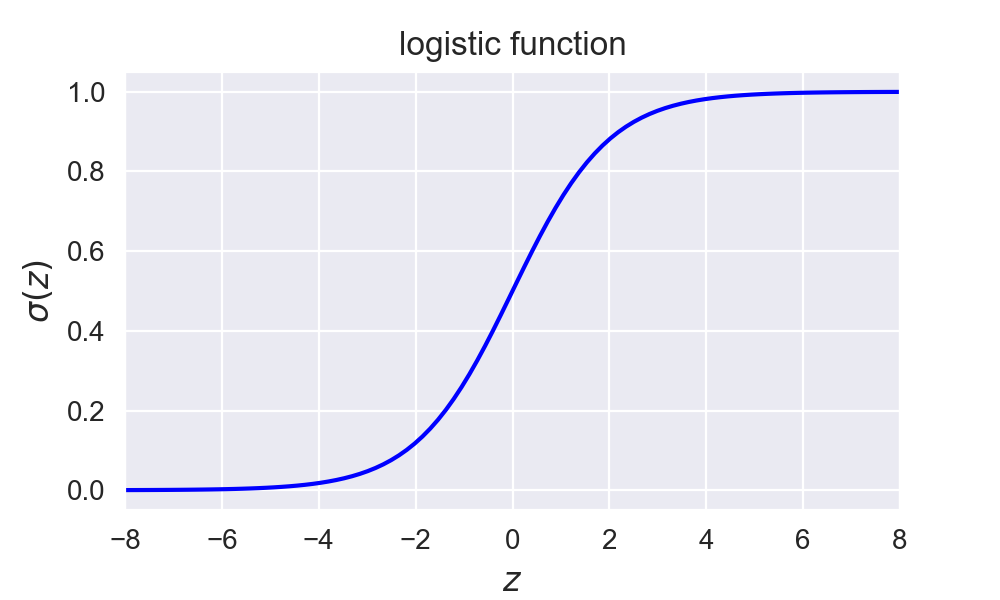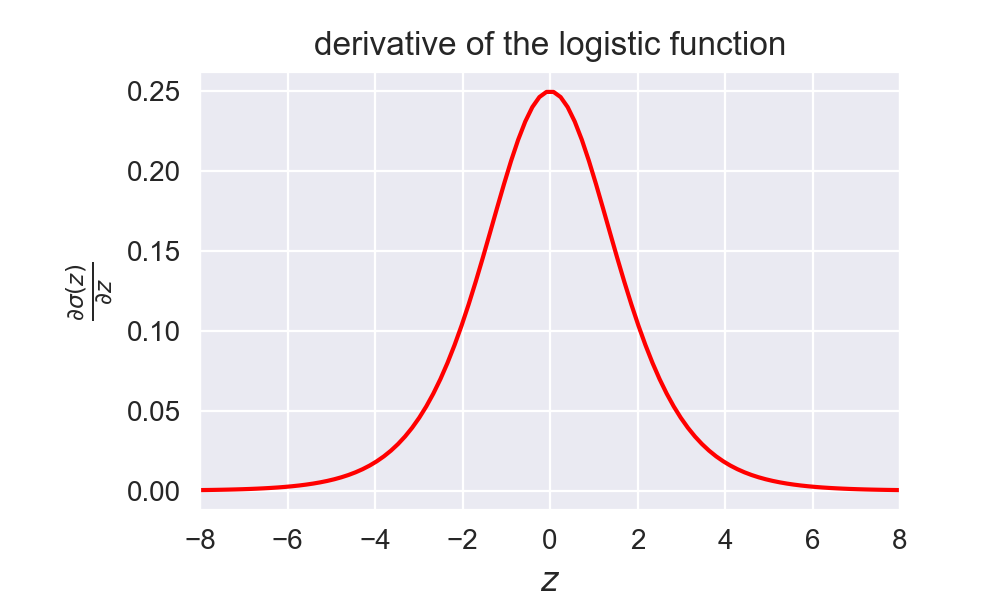# Logistic classification with cross-entropy

This tutorial will cover how to classify a binary classification problem with help of the logistic function and the cross-entropy loss function.

If we want to do classification with neural networks we want to output a probability distribution over the classes from the output targets $t$. For the classification of 2 classes $t=1$ or $t=0$ we can use the logistic function used in logistic regression . For multiclass classification there exists an extension of this logistic function called the softmax function which is used in multinomial logistic regression . The following section will explain the softmax function and how to derive it. What follows here will explain the logistic function and how to optimize it.

In :

## Logistic function ¶

The goal is to predict the target class $t$ from an input $z$. The probability $P(t=1 | z)$ that input $z$ is classified as class $t=1$ is represented by the output $y$ of the logistic function computed as $y = \sigma(z)$. The logistic function $\sigma$ is defined as: $$\sigma(z) = \frac{1}{1+e^{-z}}$$

This logistic function, implemented below as  logistic(z)  , maps the input $z$ to an output between $0$ and $1$ as is illustrated in the figure below.

We can write the probabilities that the class is $t=1$ or $t=0$ given input $z$ as:

$$\begin{split} P(t=1| z) & = \sigma(z) = \frac{1}{1+e^{-z}} \\ P(t=0| z) & = 1 - \sigma(z) = \frac{e^{-z}}{1+e^{-z}} \end{split}$$

Note that input $z$ to the logistic function corresponds to the log odds ratio of $P(t=1|z)$ over $P(t=0|z)$.

$$\begin{split} \log \frac{P(t=1|z)}{P(t=0|z)} & = \log \frac{\frac{1}{1+e^{-z}}}{\frac{e^{-z}}{1+e^{-z}}} = \log \frac{1}{e^{-z}} \\ & = \log(1) - \log(e^{-z}) = z \end{split}$$

This means that the logg odds ratio $\log(P(t=1|z)/P(t=0|z))$ changes linearly with $z$. And if $z = x \cdot w$ as in neural networks, this means that the logg odds ratio changes linearly with the parameters $w$ and input samples $x$.

In :
def logistic(z):
"""Logistic function."""
return 1 / (1 + np.exp(-z))

In :### Derivative of the logistic function ¶

Since neural networks typically use gradient based opimization techniques such as gradient descent it is important to define the derivative of the output $y$ of the logistic function with respect to its input $z$. ${\partial y}/{\partial z}$ can be calculated as:

$$\frac{\partial y}{\partial z} = \frac{\partial \sigma(z)}{\partial z} = \frac{\partial \frac{1}{1+e^{-z}}}{\partial z} = \frac{-1}{(1+e^{-z})^2} \cdot e^{-z} \cdot -1 = \frac{1}{1+e^{-z}} \frac{e^{-z}}{1+e^{-z}}$$

And since $1 - \sigma(z)) = 1 - {1}/(1+e^{-z}) = {e^{-z}}/(1+e^{-z})$ this can be rewritten as:

$$\frac{\partial y}{\partial z} = \frac{1}{1+e^{-z}} \frac{e^{-z}}{1+e^{-z}} = \sigma(z) \cdot (1- \sigma(z)) = y (1-y)$$

This derivative is implemented as  logistic_derivative(z)  and is plotted below.

In :
def logistic_derivative(z):
"""Derivative of the logistic function."""
return logistic(z) * (1 - logistic(z))

In :### Cross-entropy loss function for the logistic function ¶

The output of the model $y = \sigma(z)$ can be interpreted as a probability $y$ that input $z$ belongs to one class $(t=1)$, or probability $1-y$ that $z$ belongs to the other class $(t=0)$ in a two class classification problem. We note this down as: $P(t=1| z) = \sigma(z) = y$.

The neural network model will be optimized by maximizing the likelihood that a given set of parameters $\theta$ of the model can result in a prediction of the correct class of each input sample. The parameters $\theta$ transform each input sample $i$ into an input to the logistic function $z_{i}$. The likelihood maximization can be written as:

$$\underset{\theta}{\text{argmax}}\; \mathcal{L}(\theta|t,z) = \underset{\theta}{\text{argmax}} \prod_{i=1}^{n} \mathcal{L}(\theta|t_i,z_i)$$

The likelihood $\mathcal{L}(\theta|t,z)$ can be rewritten as the joint probability of generating $t$ and $z$ given the parameters $\theta$: $P(t,z|\theta)$. Since $P(A,B) = P(A|B)P(B)$ this can be written as:

$$P(t,z|\theta) = P(t|z,\theta)P(z|\theta)$$

Since we are not interested in the probability of $z$ we can reduce this to: $\mathcal{L}(\theta|t,z) = P(t|z,\theta) = \prod_{i=1}^{n} P(t_i|z_i,\theta)$. Since $t_i$ is a Bernoulli variable , and the probability $P(t| z) = y$ is fixed for a given $\theta$ we can rewrite this as:

$$\begin{split} P(t|z) & = \prod_{i=1}^{n} P(t_i=1|z_i)^{t_i} \cdot (1 - P(t_i=1|z_i))^{1-t_i} \\ & = \prod_{i=1}^{n} y_i^{t_i} \cdot (1 - y_i)^{1-t_i} \end{split}$$

Since the logarithmic function is a monotone increasing function we can optimize the log-likelihood function $\underset{\theta}{\text{argmax}}\; \log \mathcal{L}(\theta|t,z)$. This maximum will be the same as the maximum from the regular likelihood function. The benefit of using the log-likelihood is that it can prevent numerical underflow when the probabilities are low. The log-likelihood function can be written as:

$$\begin{split} \log \mathcal{L}(\theta|t,z) & = \log \prod_{i=1}^{n} y_i^{t_i} \cdot (1 - y_i)^{1-t_i} \\ & = \sum_{i=1}^{n} t_i \log(y_i) + (1-t_i) \log(1 - y_i) \end{split}$$

Minimizing the negative of this function (minimizing the negative log likelihood) corresponds to maximizing the likelihood. This error function $\xi(t,y)$ is typically known as the cross-entropy error function (also known as log-loss):

$$\begin{split} \xi(t,y) & = - \log \mathcal{L}(\theta|t,z) \\ & = - \sum_{i=1}^{n} \left[ t_i \log(y_i) + (1-t_i)\log(1-y_i) \right] \\ & = - \sum_{i=1}^{n} \left[ t_i \log(\sigma(z)) + (1-t_i)\log(1-\sigma(z)) \right] \end{split}$$

This function looks complicated but besides the previous derivation there are a couple of intuitions why this function is used as a loss function for logistic regression. First of all it can be rewritten as:

$$\xi(t_i,y_i) = \begin{cases} -\log(y_i) & \text{if } t_i = 1 \\ -\log(1-y_i) & \text{if } t_i = 0 \end{cases}$$

Which in the case of $t_i=1$ is $0$ if $y_i=1$ $(-\log(1)=0)$ and goes to infinity as $y_i \rightarrow 0$ $(\underset{y \rightarrow 0}{\text{lim}}{(-\log(y))} = +\infty)$. The reverse effect is happening if $t_i=0$.
So what we end up with is a loss function that is $0$ if the probability to predict the correct class is $1$ and goes to infinity as the probability to predict the correct class goes to $0$.

Notice that the loss function $\xi(t,y)$ is equal to the negative log probability that $z$ is classified as its correct class:
$$\begin{split} -\log(P(t=1| z)) &= -\log(y) \\ -\log(P(t=0| z)) &= -\log(1-y) \end{split}$$

By minimizing the negative log probability, we will maximize the log probability. And since $t$ can only be $0$ or $1$, we can write $\xi(t,y)$ as:

$$\xi(t,y) = -t \log(y) - (1-t) \log(1-y)$$

Which will give $\xi(t,y) = - \sum_{i=1}^{n} \left[ t_i \log(y_i) + (1-t_i)\log(1-y_i) \right]$ if we sum over all $n$ samples.

Another reason to use the cross-entropy function is that in simple logistic regression this results in a convex loss function, of which the global minimum will be easy to find. Note that this is not necessarily the case anymore in multilayer neural networks.

#### Derivative of the cross-entropy loss function for the logistic function ¶

The derivative ${\partial \xi}/{\partial y}$ of the loss function with respect to its input can be calculated as:

$$\begin{split} \frac{\partial \xi}{\partial y} &= \frac{\partial (-t \log(y) - (1-t) \log(1-y))}{\partial y} = \frac{\partial (-t \log(y))}{\partial y} + \frac{\partial (- (1-t) \log(1-y))}{\partial y} \\ & = -\frac{t}{y} + \frac{1-t}{1-y} = \frac{y-t}{y(1-y)} \end{split}$$

This derivative will give a nice formula if it is used to calculate the derivative of the loss function with respect to the inputs of the classifier ${\partial \xi}/{\partial z}$ since the derivative of the logistic function is ${\partial y}/{\partial z} = y (1-y)$:

$$\frac{\partial \xi}{\partial z} = \frac{\partial y}{\partial z} \frac{\partial \xi}{\partial y} = y (1-y) \frac{y-t}{y(1-y)} = y-t$$

In :
Python: 3.7.0
numpy: 1.15.2
matplotlib: 3.0.0
seaborn: 0.9.0


This is the first part of a 2-part tutorial on classification models trained by cross-entropy:

This post at peterroelants.github.io is generated from an IPython notebook file. Link to the full IPython notebook file